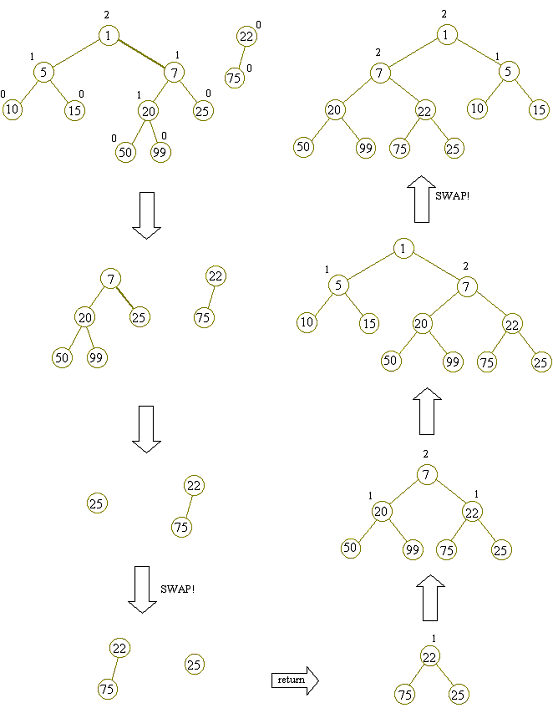# 左偏树/斜堆——可并堆详解

H ← Merge(H1,H2)
Merge( ) 构造并返回一个包含H1和H2所有元素的新堆H。

。。原文里有一些没什么卵用的引理和性质我就不说了，显得冗余，看着都烦

if(dist[p[x].l]<dist[p[x].r])             swap(dist[p[x].l],dist[p[x].r]);

if(!x)  return y;
if(!y)  return x;

x和y为当前要合并的两个子树inline int merge(int x,int y)
{
if(!x)  return y;
if(!y)  return x;
if(p[x].val>p[y].val||(p[x].val==p[y].val&&x>y))    swap(x,y);
int son=merge(p[x].r,y);
p[x].r=son;
if(dist[p[x].l]<dist[p[x].r])   swap(dist[p[x].l],dist[p[x].r]);
dist[x]=dist[son]+1;
return x;
}

inline int get(int x)
{
return x==p[x].father?x:p[x].father=get(p[x].father);
}

    int tx=get(x);
printf("%d\n",p[tx].val);
alr[tx]=1;
int the_aci=merge(p[tx].l,p[tx].r);
p[tx].father=the_aci;//tx节点已经被删除，但是他的儿子们在get函数中需要通过tx来更新
p[the_aci].father=the_aci;

alr[tx]表示tx节点已经被删除

CODE：

#include<cstdio>
#include<algorithm>
#include<iostream>
#include<cmath>
using namespace std;
struct point
{
int l,r,father,val;
}p;
bool alr;//记录节点是否被删除
int dist;
int n,m;
inline int get(int x)
{
return x==p[x].father?x:p[x].father=get(p[x].father);
}
inline int merge(int x,int y)
{
if(!x)  return y;
if(!y)  return x;
if(p[x].val>p[y].val||(p[x].val==p[y].val&&x>y))    swap(x,y);
int son=merge(p[x].r,y);
p[x].r=son;
if(dist[p[x].l]<dist[p[x].r])   swap(dist[p[x].l],dist[p[x].r]);
dist[x]=dist[son]+1;
return x;
}
int main()
{
scanf("%d",&n);
for(int i=1;i<=n;i++)
{
scanf("%d",&p[i].val),p[i].father=i;
}
scanf("%d",&m);
for(int i=1;i<=m;i++)
{
char kind;
scanf("%s",kind);
if(kind=='M')
{
int x,y;
scanf("%d%d",&x,&y);
if(alr[x]||alr[y])  continue;
int tx=get(x),ty=get(y);
if(tx==ty)  continue;
else
{
int the_aci=merge(tx,ty);
p[tx].father=p[ty].father=p[the_aci].father;
}
}
else
{
int x;
scanf("%d",&x);

if(alr[x])
{
printf("0\n");
continue;
}else
{
int tx=get(x);
printf("%d\n",p[tx].val);
alr[tx]=1;
int the_aci=merge(p[tx].l,p[tx].r);
p[tx].father=the_aci;//tx节点已经被删除，但是他的儿子们在get函数中需要通过tx来更新
p[the_aci].father=the_aci;
}
}
}
}

if(dist[p[x].l]<dist[p[x].r])   swap(dist[p[x].l],dist[p[x].r]);
dist[x]=dist[son]+1;©️2020 CSDN 皮肤主题: 大白 设计师:CSDN官方博客